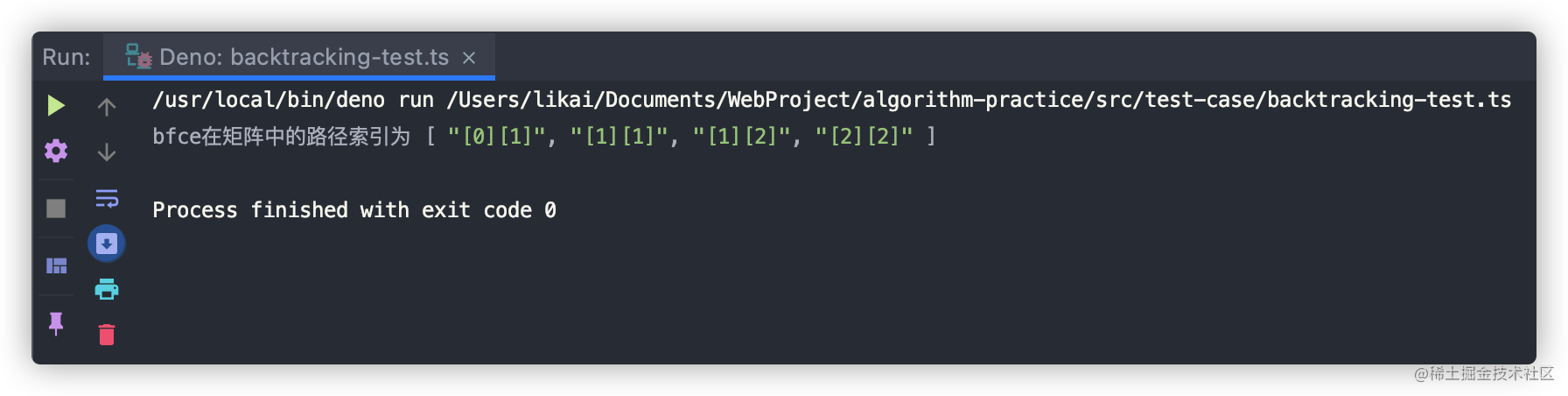• 累计撰写 149 篇文章
• 累计创建 25 个标签
• 累计收到 71 条评论

### 目 录CONTENT# 回溯算法 - 寻找矩阵中的路径2021-07-14 / 0 评论 / 1 点赞 / 80 阅读 / 3,387 字

## 实现思路

### 举例分析

a  b  t  g
c  f  c  s
j  d  e  h


• 0,0 位置的元素是a，与目标值不匹配，继续寻找0,1位置
• 0,1 位置的元素是是b，与目标值匹配，继续查找第2个字符f
• 更新寻找方向，向下查找
• 1,1 位置的元素是f，与目标值匹配，继续查找第3个字符c
• 更新寻找方向，向下查找
• 2,1 位置的元素是d，与目标值不匹配，回到上一步1,1位置
• 更新寻找方向，向上查找，
• 0,0位置的值已经寻找过了，回到上一步1,1位置
• 更新寻找方向，向右查找
• 1,2 位置的元素是c，与目标值匹配，继续查找第4个字符e
• 更新寻找方向，向下查找
• 2,2 位置的元素是e，与目标值匹配，所有字符寻找完毕，该路径存在与矩阵中

• [2,2]位置
• [1,2]位置
• [1,1]位置
• [0,1]位置

### 思路分析

• 寻找一个切入点，从第一个字符开始寻找其在矩阵中的位置
• 进入矩阵后，每一步都会有4个移动方向：下、上、右、左
• 每移动一个方向，都会判断移动后位置的值是否与当前要查找的字符是否相等
• 如果相等，则标识当前位置的元素为已访问状态，沿着四个移动方向继续寻找下一个字符
• 如果不相等，则回到上一步的位置点，尝试其他的三个方向是否有匹配的元素
• 重复步骤3，直至所有匹配字符的四个方向都被移动
• 字符串中的全部字符都被找到后，则取出每一步的正确索引位置将其保存起来
• 四个方向都被移动后，仍未找到与字符所匹配的元素，则证明该字符串不存在于矩阵中

## 实现代码

• 主函数，用于参数规则判断、寻找切入点、返回找到的路径
• 寻找路径函数，用于在矩阵中寻找每一个字符

### 主函数

• 我们需要先对参数进行判空
• 遍历矩阵从0,0位置开始寻找路径
• 路径找到则返回路径索引，否则返回目标路径不存在

export default class Backtracking {
// 目标路径在矩阵中的索引
private readonly pathIndex: Array<string>;

constructor() {
this.pathIndex = [];
}

public findMatrixPath(
matrix: Array<Array<string>>,
target: string
): Array<string> {
if (target === "") {
this.pathIndex.push("参数错误: 目标路径为空");
return this.pathIndex;
}
for (let i = 0; i < matrix.length; i++) {
for (let j = 0; j < matrix[i].length; j++) {
if (this.findPath(matrix, target, i, j, 0)) {
return this.pathIndex;
}
}
}
// 未找到
this.pathIndex.push("目标路径不存在于矩阵中");
return this.pathIndex;
}
}


### 寻找路径函数

• 首先，我们需要判断下要寻找的行、列是否超越矩阵的界限
• 矩阵中要寻找的行、列位置的元素与要寻找的字符不相等则直接返回false
• 判断所有字符是否都查找完成
• 完成的话则存储行、列索引，返回true
• 未完成则保存当前行、列处的值、修改该位置的值为.用于标识为已访问状态
• 从当前坐标点位置沿着其四个方向：下、上、右、下进行查找
• 查找完成后保存已找到字符的坐标点，还原当前位置所保存的值

  private findPath(
matrix: Array<Array<string>>,
target: string,
row: number,
col: number,
index: number
): boolean {
// 边界条件判断
//  1. 行、列值越界直接返回false
//  2. matrix[row][col]位置的元素与当前要查找的字符不等，证明这个路径走不通
if (
row >= matrix.length ||
row < 0 ||
col >= matrix.length ||
col < 0 ||
matrix[row][col] != target[index]
) {
return false;
}
// 所有字符都已查找完成
if (index === target.length - 1) {
// 保存最后一个字符在矩阵中的坐标
this.pathIndex.unshift([${row}][${col}]);
return true;
}
// 保存当前坐标值
const tmp = matrix[row][col];
// 修改当前坐标的值，标识当前坐标点已经被寻找
matrix[row][col] = ".";
// 开始递归: 沿着当前坐标的上下左右4个方向查找
const res: boolean =
this.findPath(matrix, target, row + 1, col, index + 1) ||
this.findPath(matrix, target, row - 1, col, index + 1) ||
this.findPath(matrix, target, row, col + 1, index + 1) ||
this.findPath(matrix, target, row, col - 1, index + 1);
// 本轮递归完成，找到了当前要查找字符在矩阵中的位置
if (res) {
// 保存当前要查找字符的坐标点
this.pathIndex.unshift([${row}][${col}]);
}
// 递归完成，复原当前坐标
matrix[row][col] = tmp;
return res;
}


### 编写测试用例

import Backtracking from "../Backtracking.ts";

const pathArr = [
["a", "b", "t", "g"],
["c", "f", "c", "s"],
["j", "d", "e", "h"]
];
const target = "bfce";
const backtracking = new Backtracking();
const findResult = backtracking.findMatrixPath(pathArr, target);
console.log(\${target}在矩阵中的路径索引为, findResult);## 写在最后

• 文中如有错误，欢迎在评论区指正，如果这篇文章帮到了你，欢迎点赞和关注😊
• 本文首发于掘金，未经许可禁止转载💌
1